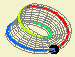## Paths on a surface### Mobius band - get to know the surface

$$\DeclareMathOperator{\sen}{sen}$$[see app]

We can think of Moebius strip as a surface obtained from gluing two parallel sides of a rectangle, after twisting once one of the sides. The sides that are not glued will form a single closed curve.

Therefore, there is an identification between the points of the rectangle and the points of the strip.

$(u,v)\rightarrow\left(\cos(u)\left(r+v\cos\left(\frac{u}{2}\right)\right),\sen(u)\left(r+v\cos\left(\frac{u}{2}\right)\right),v\sen\left(\frac{u}{2}\right)\right).$ The glued points on the rectangles sides correspond to the same segment in the strip. Therefore, there is an identification (with opposite orientation) between the points on the sides with opposite sides, as illustrated by their coloring.

Drawing a curve in the strip that crosses the glued line, corresponds, in the rectangle, to "going out" from one side and "coming in" from the other, from the corresponding point (at a symmetrical hight).

The longitude and latitude of the points give us the coordinates of its location over the surface. The latitude varies in an interval $$I$$, closed and symmetrical, of $$\mathbb{R}$$. The longitude is the measure of an angle (in radians) "around" the surface. For the identification of a point over the surface we only need the longitude to vary in the interval $$\left[0,2\pi\right[$$, as it covers the surface completely.

However, to allows us to parameterize continuously the curve over the surface, we can extend this interval to an interval $$J$$ with the following identification: $\begin{array}{ccc} J\times I & \rightarrow & \left[0,2\pi\right[\times I\\ (u,v) & \rightarrow & (\mbox{Mod}\left[u,2\pi\right],-v) \end{array}$

This extension materializes in the apps through a change on scale, allowing a better description of the curves that go around the cylinder several times.

A lift of the paths in a Moebius strip can be extended to an infinite strip on the plane, allowing for a simultaneous study of the curves in the Moebius strip independently of the number of times around it. We could consider, analogously, an infinitude of copies of the initial rectangle, properly aligned and oriented, with which we can say that each point of the plane projects into a point of the Moebius strip, and that each point of the Moebius strip lifts into an infinitude of points in the strip of the plane, one in each of the rectangles.